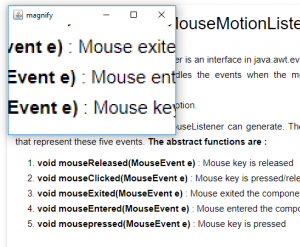# JSwing | Create a Magnifying tool using Java Robot

Java Robot is a part of Java AWT (Abstract Window Toolkit ) package . Java Robot is used to generate native system input events for the purposes of test automation, self-running demos, and other applications where control of the mouse and keyboard is needed. The purpose of using Java Robot is to gain the control of input events such as mouse, keyboard etc.

In this article we will create a Magnifying toolusing JAVA Robot .

Methods used :

1. getPointerInfo() : Returns a PointerInfo instance that represents the current location of the mouse pointer.
2. getLocation() : returns a point instance that represents location
3. createScreenCapture(Rectangle r) : captures a part of screen which is within the rectangle r.
4. drawImage(Image i, int x, int y, ImageObserver o) : draws an image at x, y position on the screen specifying an image observer
5. drawImage(Image i, int x, int y,,int w, int h, ImageObserver o) : draws an image at x, y position and specified width and height on the screen specifying an image observer

Java program to create a Magnifying tool using Java Robot

 `// Java program to create a Magnifying tool ` `// using Java Robot ` ` `  `import` `java.awt.event.*; ` `import` `javax.swing.*; ` `import` `java.awt.*; ` `class` `magnify ``extends` `JFrame { ` ` `  `    ``// object ` `    ``static` `magnify m; ` ` `  `    ``// image ` `    ``Image i; ` ` `  `    ``// default constrcutor ` `    ``magnify() ` `    ``{ ` `        ``// create a frame ` `        ``super``(``"magnify"``); ` ` `  `        ``// set size of frame ` `        ``setSize(``200``, ``220``); ` `        ``show(); ` ` `  `        ``// function to magnify the image ` `        ``work(); ` `    ``} ` ` `  `    ``// main function ` `    ``public` `static` `void` `main(String args[]) ` `    ``{ ` ` `  `        ``// object of class ` `        ``m = ``new` `magnify(); ` `    ``} ` ` `  `    ``public` `void` `work() ` `    ``{ ` `        ``try` `{ ` `            ``// create a robot ` `            ``Robot r = ``new` `Robot(); ` ` `  `            ``// while the frame is visible ` `            ``while` `(isVisible()) { ` `                ``// get the position of mouse ` `                ``Point p = MouseInfo.getPointerInfo().getLocation(); ` ` `  `                ``// create a screen capture around the mouse pointer ` `                ``i = r.createScreenCapture(``new` `Rectangle((``int``)p.getX() - ``30``, ` `                                                        ``(``int``)p.getY() - ``30``, ``150``, ``150``)); ` ` `  `                ``// repaint the conatiner ` `                ``repaint(); ` `            ``} ` `            ``// exit the program ` `            ``System.exit(``0``); ` `        ``} ` `        ``catch` `(Exception e) { ` `            ``System.err.println(e.getMessage()); ` `        ``} ` `    ``} ` ` `  `    ``// paint function ` `    ``public` `void` `paint(Graphics g) ` `    ``{ ` ` `  `        ``// draw the image ` `        ``g.drawImage(i, ``0``, ``0``, ``300``, ``300``, ``this``); ` `    ``} ` `} `

Output :Attention reader! Don’t stop learning now. Get hold of all the important DSA concepts with the DSA Self Paced Course at a student-friendly price and become industry ready.

My Personal Notes arrow_drop_upCheck out this Author's contributed articles.

If you like GeeksforGeeks and would like to contribute, you can also write an article using contribute.geeksforgeeks.org or mail your article to contribute@geeksforgeeks.org. See your article appearing on the GeeksforGeeks main page and help other Geeks.

Please Improve this article if you find anything incorrect by clicking on the "Improve Article" button below.

Article Tags :
Practice Tags :

1

Please write to us at contribute@geeksforgeeks.org to report any issue with the above content.